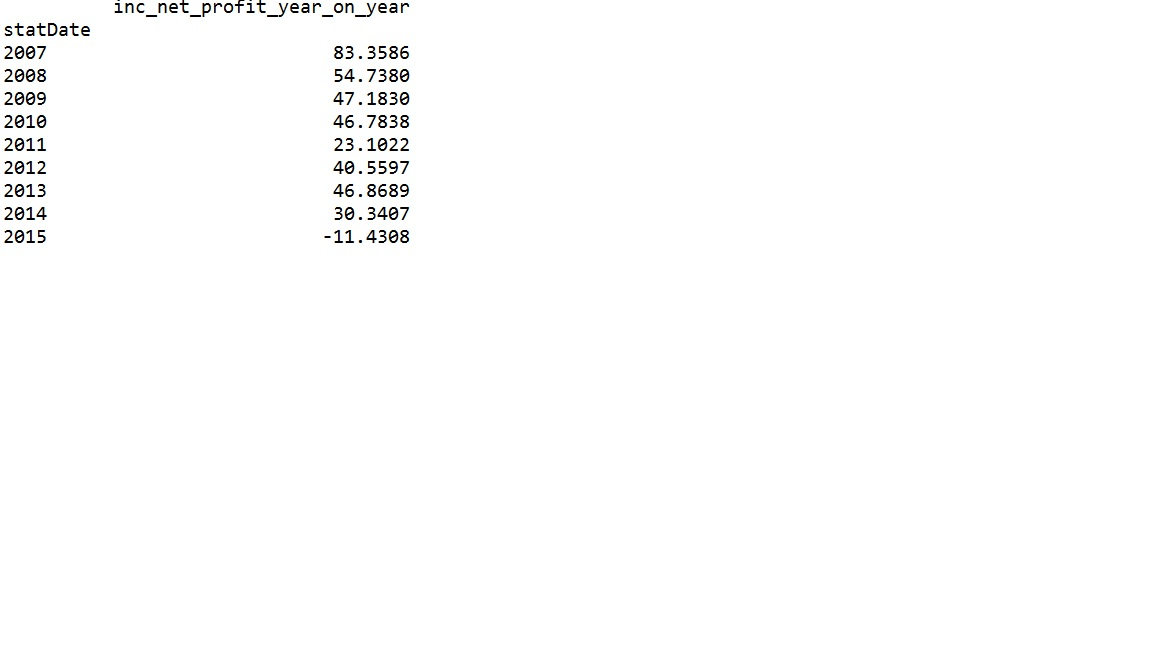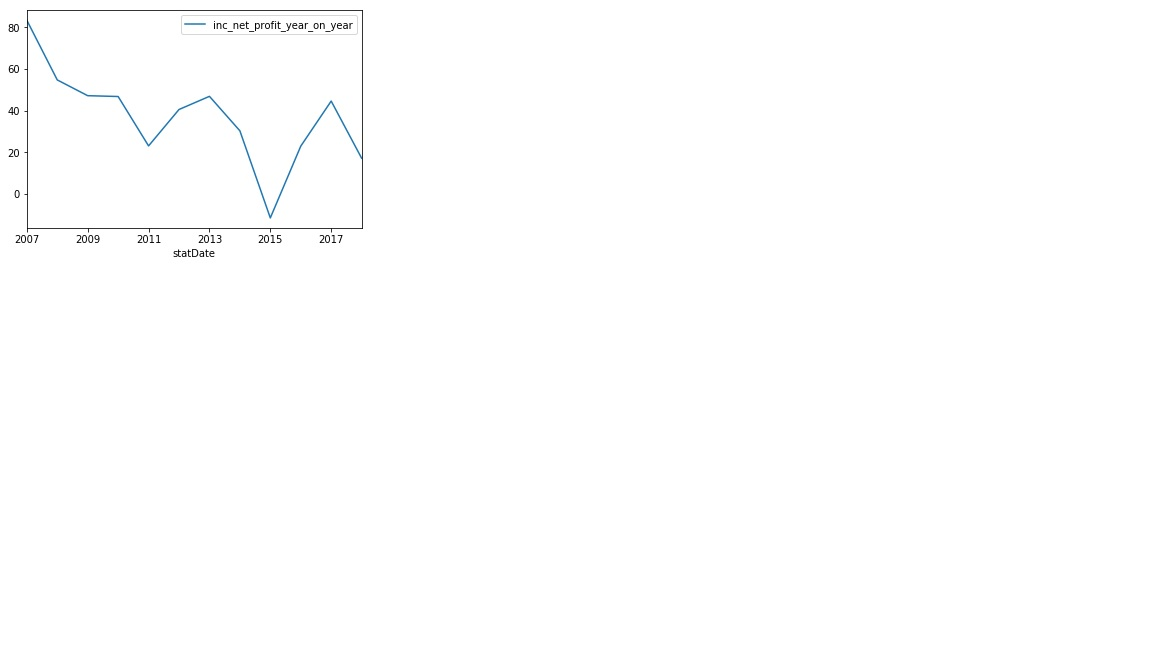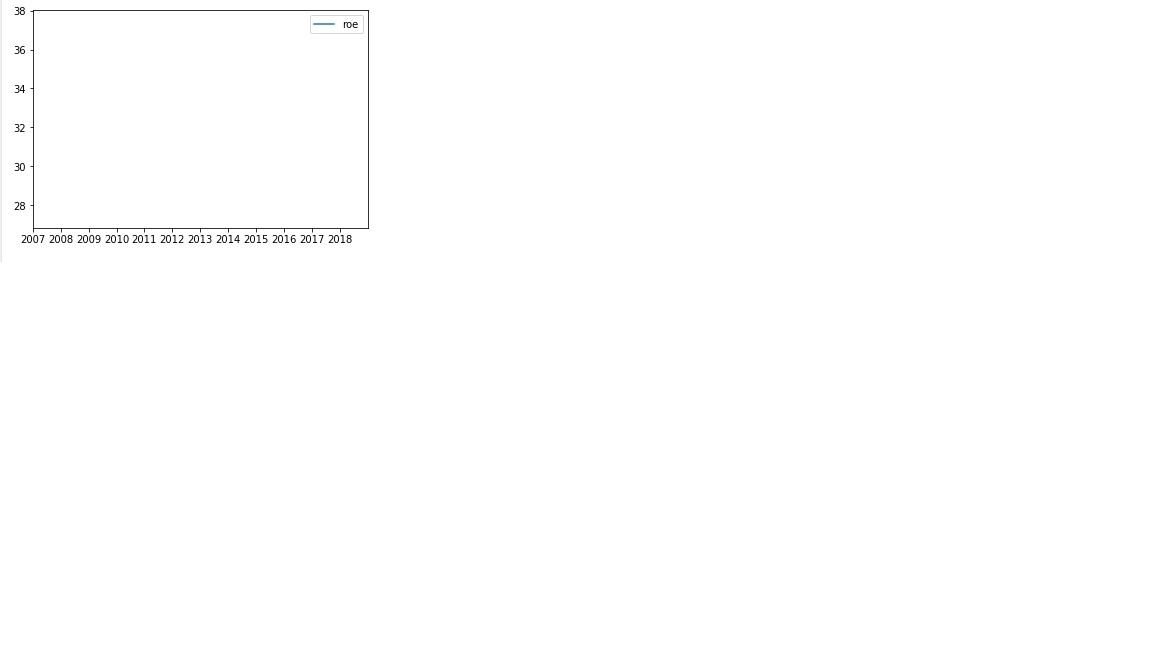ply作图坐标轴刻度问题df1.plot(xticks = np.arange(2007, 2019, 1),xlim = (2007,2019))1个回答

tmp=df.plt()
tmp.set_xticklabels(list(range(2007,2019)))